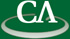## On Fulton's Algorithm for Computing Intersection Multiplicities.

### Paul Vrbik, Department of Computer Science, University of Western Ontario

July 9th at 1pm in K9509.

```As pointed out by Fulton in his book: Intersection Theory, the intersection
multiplicities of two plane curves V(f) and V(g) satisfy a series of 7 properties
which uniquely define the intersection multiplicity, I(p; f, g), at each point
p in V(f, g). Moreover, the proof of this remarkable fact is constructive,
yielding what we call Fulton's Algorithm. This construction, however,
does not generalize to n polynomials f1,...,fn and does not account
for points that lie outside the (usually rational) coefficient field.

In this work, we adapt Fulton's Algorithm so that it can work at any
point of V(f, g), rational or not. In addition, we propose algorithmic criteria
for reducing the case of n variables to the bivariate one.
Implementation details and experimental results are reported.

This is joint work with Steffen Marcus and Marc Moreno Maza.
```